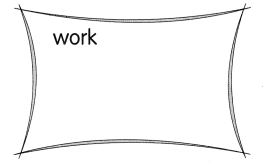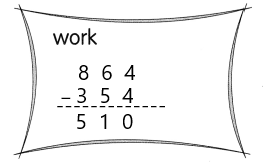# Math in Focus Grade 2 Chapter 3 Practice 1 Answer Key Subtraction Without Regrouping

Go through the Math in Focus Grade 2 Workbook Answer Key Chapter 3 Practice 1 Subtraction Without Regrouping to finish your assignments.

## Math in Focus Grade 2 Chapter 3 Practice 1 Answer Key Subtraction Without Regrouping

Subtract.

Question 1.
432 – 221 = ?
Subtract the ones.
2 ones – 1 one = ___ one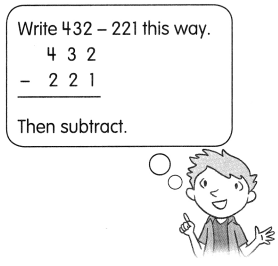Subtract the tens.
3 tens – 2 tens = ____ ten
Subtract the hundreds.
4 hundreds – 2 hundreds = ___ hundreds
432 – 221 = ____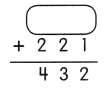432 – 221 = 211,
211+221 = 432.

Explanation:
Given that 432 – 221 which is 211. So to check the answer we will perform addition, which is
211+221 = 432.
Subtract the tens.
3 tens – 2 tens = 1 ten
Subtract the hundreds.
4 hundreds – 2 hundreds = 2 hundreds
432 – 221 = 211.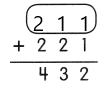Question 2.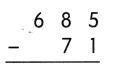685-71 = 614,
614+71 = 685.

Explanation:
Given that 685-71 which is 614. So to check the answer we will perform addition, which is
614+71 = 685.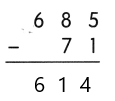Question 3.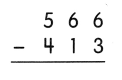566-413 = 153,
413+153 = 566.

Explanation:
Given that 566-413 which is 153. So to check the answer we will perform addition, which is
413+153 = 566.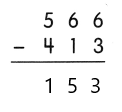Question 4.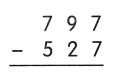797-527 = 270,
270+527 = 797.

Explanation:
Given that 797-527 which is 270. So to check the answer we will perform addition, which is
270+527 = 797.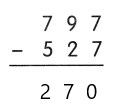Question 5.
999 – 693 = ___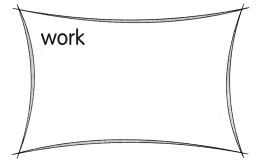999-693 = 306,
306+693 = 999.

Explanation:
Given that 999-693 which is 306. So to check the answer we will perform addition, which is
306+693 = 999.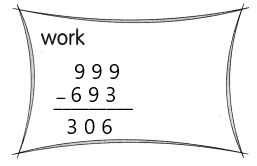Question 6.
864 – 354 = ___